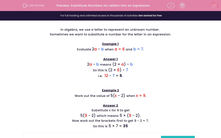# Substitute Numbers for Letters into an Expression

In this worksheet, students will substitute numbers in place of letters to evaluate the given expressions.This content is premium and exclusive to EdPlace subscribers.Key stage:  KS 2

Curriculum topic:   Maths and Numerical Reasoning

Curriculum subtopic:   Equations and Algebra

Difficulty level:#### Worksheet Overview

In algebra, we use a letter to represent an unknown number.

Sometimes we want to substitute a number for the letter in an expression.

Example 1

Evaluate 2a - b when a = 6 and b = 7.

2a - b means (2 × a) - b

So this is (2 × 6) - 7

i.e.  12 - 7 = 5.

Example 2

Work out the value of 5(x - 2) when x = 9.

Substitute x for 9 to get

5(9 - 2) which means 5 × (9 - 2).

Now work out the brackets first to get 9 - 2 = 7.

So this is 5 × 7 = 35

If this looks daunting, just take it one step at a time and write down each of the steps as you go along. If you need to look at this introduction again, just click on the red help button on the screen.

Let's get started!### What is EdPlace?

We're your National Curriculum aligned online education content provider helping each child succeed in English, maths and science from year 1 to GCSE. With an EdPlace account you’ll be able to track and measure progress, helping each child achieve their best. We build confidence and attainment by personalising each child’s learning at a level that suits them.

Get started